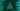# C# program to find the area of a circle## C# program to find the area of a circle :

In this post, we will learn how to find the area of a circle in C sharp. We will take the radius as input from the user and calculate the area. With this program, you will learn how to take user inputs in C sharp and how to do mathematical calculations.

### Formula to find the area of a circle:

Below formula is used to calculate the circle area:

``π * r * r``

Where, r is the radius and π is the mathematical constant Pi. We will take the value of radius from the user as input. For π, we can either define a variable and assign it upto a specific decimal place or we can use Math.PI constant that is available in C#.

### C# program:

Below is the complete program to calculate circle area in C#:

``````using System;

public class Program
{
public static void Main()
{
double area;

Console.WriteLine("Enter the value of radius: ");

Console.WriteLine("Area of the circle is : "+ Math.Round(area,2));
}
}``````

## Explanation:

• radius is an int value to keep the radius of the circle.
• area is a double to save the area of the circle.
• We are using int.Parse to parse the user input number i.e. the radius.
• The final area is stored in the double area
• Finally, we are writing down the area of the triangle.

### Sample output:

``````Enter the value of radius:
10
Area of the circle is :314.16``````